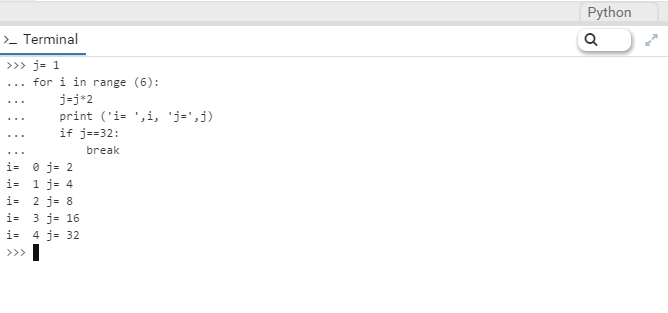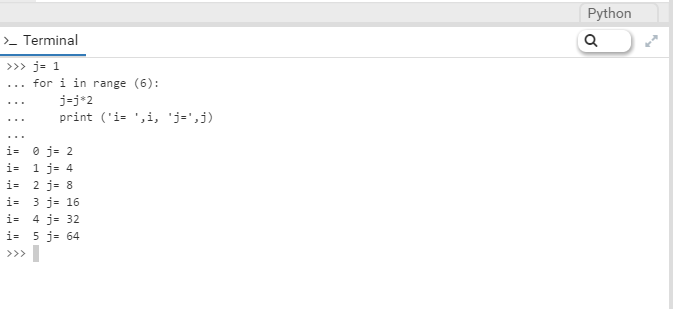# Using Break condition in Python

This article will show a step by step guide on how use Break in Python

In many use cases, or due to certain business rules, we need to exit a loop in any programming language. Or, we need to stop a particular loop and continue with the rest of the code.

This article will help you to understand the use of a break in Python which is mainly used to exit the loops in python programming.

To achieve this we need to use “break” keyword while running a loop.

Let’s take a very simple example of how to use a break in Python programming language.

Example :

``````j= 1
for i in range (6):
j=j*2
print ('i= ',i, 'j=',j)
if j==32:
break
``````

If we execute the above code in any Python interpreter, then we will see the following result :So let’s try to understand the code without adding the break statement.

``````j= 1
for i in range (6):
j=j*2
print ('i= ',i, 'j=',j)

``````

Now we see the code execute without any break. The loop starts from 1 till 5 as we have provided the range as “6” so starting from 0 till 5 the loop will execute :If we compare the code with a break keyword, we have given an argument as if the result of “j” will be 32, then end the execution of the code so that the loop will run till range 5, as at this point the result of “j” is “32”.

This article was to explain and show you a simple programme on how to use a break statement in Python. I hope I’ve achieved just that.

Thank you for reading my blog, the reference is taken from one of my article that was published by Experts-Exchange.

Please feel free to leave me some feedback or to suggest any future topics.

1.mouniyoga says: